# NCERT Solutions for Class 9 Science Chapter 4 Structure of the Atom

These NCERT Solutions for Class 9 Science Chapter 4 Structure of the Atom Questions and Answers are prepared by our highly skilled subject experts to help students while preparing for their exams.

## Structure of the Atom NCERT Solutions for Class 9 Science Chapter 4

### Class 9 Science Chapter 4 Structure of the Atom InText Questions and Answers

Question 1.
What are canal rays?
Canal rays are positively charged radiations. These rays consist of positively charged particles known as protons. They were discovered by Goldstein in 1886.

Question 2.
If an atom contains one electron and one proton, will it cany any charge or not?
An electron is a negatively charged; particle, whereas a proton is a positively charged; particle. The magnitude of their charges is equal. Therefore, an atom containing one electron and one proton will not carry any charge. Thus, it will be a neutral atom.

Question 3.
Name the three sub-atomic particles of an atom.
The three sub-atomic particles of an atom are:

1. Protons
2. Electrons
3. Neutrons

Question 4.
Helium atom has an atomic mass of 4 u and two protons in its nucleus. How many neutrons does it have?
A helium atom has two neutrons. The mass of an atom is the sum of the masses of protons and neutrons present in its nucleus. Since helium atom has two protons, mass contributed by the two protons is (2 × 1) u = 2 u. Then, the remaining mass (4 – 2) u = 2 u is contributed $$\frac{2 \mathrm{u}}{1 \mathrm{u}}$$ = 2 by neutrons.

Question 5.
Draw a sketch of Bohr’s model of an atom with three shells.
Bohr’s model of an atom with three shellsQuestion 6.
What do you think would be the observation if the a-particle scattering experiment is carried out using a foil of a metal other than gold?
If the a-scattering experiment is carried out using a foil of a metal rather than gold, there would be no change in the observation. In the a- scattering experiment, a gold foil was taken because gold is malleable and a thin foil of gold can be easily made. It is difficult to make such foils from other metals.

Question 7.
Write the distribution of electrons in carbon and sodium atoms?
The total number of electrons in a carbon atom is 6. The distribution of electrons in carbon atom is given by:
First orbit or K-shell = 2 electrons
Second orbit or L-shell = 4 electrons
Or, we can write the distribution of electrons in a carbon atom as 2, 4.

The total number of electrons in a sodium atom is 11. The distribution of electrons in sodium atom is given by:
First orbit or K-shell = 2 electrons
Second orbit or L-shell = 8 electrons
Third orbit or M-shell = 1 electron
Or, we can write the distribution of electrons in a sodium atom as 2, 8, 1.

Question 8.
If K and L shells of an atom are full, then what would be the total number of electrons in the atom?
The maximum number of electrons that can occupy K and L-shells of an atom are 2 and 8 respectively. Therefore, if K and L-shells of an atom are full, then the total number of electrons in the atom would be (2 + 8) = 10 electrons.

Question 9.
If number of electrons in an atom is 8 and number of protons is also 8, then (i) what is the atomic number of the atom and (ii) what is the charge on the atom?
(i) The atomic number is equal to the number of protons. Therefore, the atomic number of the atom is 8.
(ii) Since the number of both electrons and protons is equal, therefore, the charge on the atom is 0.

Question 10.
With the help of Table 4.1, find out the mass number of oxygen and sulphur atom.
Mass number of oxygen = Number of protons + Number of neutrons
= 8 + 8
= 16
Mass number of sulphur=Number of protons + Number of neutrons
= 16 + 16
= 32

Question 11.
For the symbol H, D and T tabulate three sub-atomic particles found in each of them.

 Symbol Proton Neutron Electron H 1 0 1 D 1 1 1 T 1 2 1

Question 12.
Write the electronic configuration of any one pair of isotopes and isobars.
Two isoto of carbon are $$\frac {12}{6}$$C and $$\frac {14}{6}$$C.
The electronic configuration of $$\frac {12}{6}$$C is 2, 4.
The electronic configuration of $$\frac {14}{6}$$C is 2, 4.
[Isotopes have the same electronic configuration]
$$\frac {40}{20}$$Ca and $$\frac {40}{18}$$Ar are a pair of isobars
The electronic configuration of $$\frac {40}{20}$$Ca is 2, 8, 8, 2.
The electronic configuration of $$\frac {40}{18}$$Ar is 2, 8, 8.

### Class 9 Science Chapter 4 Structure of the Atom Textbook Questions and Answers

Question 1.
Compare properties of electrons, protons and neutrons.

 Electron Proton Neutron (i) Electrons are present outside the nucleus of an atom (i) Protons are present in the nucleus of an atom. (i) Neutrons are present in the of an atom (ii) Electrons are negatively charged (ii) Protons are positively charged (ii) Neutrons are neutral (iii) The mass of an electron is considered to negligible (iii) The mass of a proton is approximately 2000 times as times as the mass of an of an electron (iii) The mass of neutron is nearly equal to the mass of a proton

Question 2.
What are the limitations of J.J. Thomson’s model of the atom?
According to J.J. Thomson’s model of an atom, an atom consists of a positively charged sphere with electrons embedded in it. However, it was later found that the positively charged particles reside at the centre of the atom called the nucleus, and the electrons revolve around the nucleus.

Question 3.
What are the limitations of Rutherford’s model of the atom?
According to Rutherford’s model of an atom, electrons revolve around the nucleus in fixed orbits. But, an electron revolving in circular orbits will not be stable because during revolution, it will experience acceleration. Due to acceleration, the electrons will lose energy in the form of radiation and fall into the nucleus. In such a case, the atom would be highly unstable and collapse.

Question 4.
Describe Bohr’s model of the atom.
Bohr’s model of the atom:
Niels Bohr proposed the following postulates regarding the model of the atom.

1. Only certain orbits known as discrete orbits of electrons are allowed inside the atom.
2. While revolving in these discrete orbits, the electrons do not radiate energy.

These discrete orbits or shells are shown in the following diagram.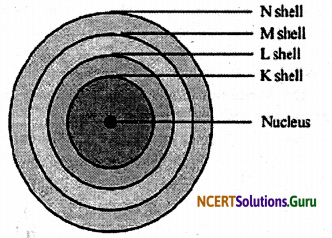The first orbit (i.e., for n = 1) is represented by letter K. Similarly, for n = 2, it is L – shell, for n = 3, it is M – shell and for n = 4, it is N – shell. These orbits or shells are also called energy levels.

Question 5.
Summarize the rules for writing of distribution of electrons in various shells for the first eighteen elements.
The rules for writing of the distribution of electrons in various shells for the first eighteen elements are given below.
(i) The maximum number of electrons that a shell can accommodate is given by the formula ‘2n2‘, where ‘n’ is the orbit number or energy level index (n = 1, 2,3..).

The maximum number of electrons present in an orbit of n = 1 is given by 2n2 = 2 × 12 = 2
Similarly, for second orbit, it is 2n2 = 2 × 22 = 8
For third orbit, it is 2n2 = 2 × 32 = 18
And so on ……
(ii) The outermost orbit can be accommodated by a maximum number of 8 electrons.
(iii) Shells are filled with electrons in a stepwise manner i.e., the outer shell is not occupied with electrons unless the inner shells are completely filled with electrons.

Question 6.
Define valency by taking examples of silicon and oxygen.
The valency of an element is the combining capacity of that element. The valency of an element is determined by the number of valence electrons present in the atom of that element.

If the number of valence electrons of the atom of an element is less than or equal to four, then the valency of that element is equal to the number of valence electrons. For example, the atom of silicon has four valence electrons. Thus, the valency of silicon is four.

On the other hand, if the number of valence electrons of the atom of an element is greater than four, then the valency of that element is obtained by subtracting the number of valence electrons from eight. For example, the atom of oxygen has six valence electrons. Thus, tire valency of oxygen is(8 – 6) i.e., two.

Question 7.
Explain with examples (i) Atomic number (ii) Mass number (iii) Isotopes and (iv) Isobars. Give any two uses of isotopes.
(i) Atomic number:
The atomic number of an element is the total number of protons present in the atom of that element. For example, nitrogen has 7 protons in its atom. Thus, the atomic number of nitrogen is 7.

(ii) Mass number:
The mass number of an element is the sum of the number of protons and neutrons present in the atom of that element. For example, tire atom of boron has 5 protons and 6 neutrons. So, the mass number of boron is 5 + 6 = 11.

(iii) Isotopes:
Isotopes are atoms of the same element having the same atomic number, but different mass numbers. For example, hydrogen has three isotopes. They are protium $$\left({ }_{1}^{1} \mathrm{H}\right)$$, deuterium $$\left({ }_{1}^{2} \mathrm{H}\right)$$, and tritium $$\left({ }_{1}^{3} \mathrm{H}\right)$$.

(iv) Isobars:
Isobars are atoms having the same mass number, but different atomic numbers i.e., isobars are atoms of different elements having the same mass number. For example, $${ }_{20}^{40} \mathrm{Ca}$$ and $${ }_{18}^{40} \mathrm{Ar}$$ andare isobars.

Two uses of isotopes are:

1. One isotope of uranium is used as a fuel in nuclear reactors.
2. One isotope of cobalt is used in the treatment of cancer.

Question 8.
Na+ has completely filled K and L shells. Explain.
An atom of Na has a total of 11 electrons. Its electronic configuration is 2,8,1. But, Na+ ion has one electron less than Na atom i.e., it has 10 electrons. Therefore, 2 electrons go to K-shell and 8 electrons go to L-shell, thereby completely filling K and L shells.

Question 9.
If bromine atom is available in tile form of, say, two isotopes $${ }_{35}^{79} \mathrm{Br}$$ (49.7%) and $${ }_{35}^{81} \mathrm{Br}$$ (50.3%), calculate the average atomic mass of bromine atom.
It is given that two isotopes of bromine are $${ }_{35}^{79} \mathrm{Br}$$(49.7%) and $${ }_{35}^{81} \mathrm{Br}$$ (50.3%). Then, the average atomic mass of bromine atom is given by:= 80.006 u
= 80 u (approx)

Question 10.
The average atomic mass of a sample of an clement X is 16.2 u. What are the
percentages of isotopes $${ }_{8}^{16} \mathrm{X}$$ and $${ }_{8}^{18} \mathrm{X}$$ in the sample?
It is given that the average atomic mass of the sample of element X is 16.2 u.
Let the percentage of isotope $${ }_{8}^{18} \mathrm{X}$$ be y%. Thus,
the percentage of isotope $${ }_{8}^{16} \mathrm{X}$$ will be (100 – y) %.

Therefore,⇒ 18y + 1600 = 1620
⇒ 2y + 1600 = 1620
⇒ 2y +1620 – 1600
⇒ 2y = 20
⇒ y = 10
Therefore, the percentage of isotope $${ }_{8}^{18} \mathrm{X}$$ is 10%.
And, the percentage of isotope $${ }_{8}^{16} \mathrm{X}$$ is (100 – 10) % = 90%.

Question 11.
If Z = 3, what would be the valency of the element? Also, name the element
By Z = 3, we mean that the atomic number of the element is 3. Its electronic configuration is 2, 1. Hence, the valency of the element is 1 (since the outermost shell has only one electron).

Therefore, the element with Z = 3 is lithium.

Question 12.
Composition of the nuclei of two atomic species X and Y are given as under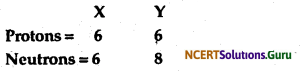Give the mass numbers of X and Y. What is the relation between the two species?
Mass number of X = Number of protons + Number of neutrons
= 6 + 6
= 12
Mass number of Y = Number of protons + Number of neutrons
= 6 + 8
= 14
These two atomic species X and Y have the same atomic number, but different mass numbers. Hence, they are isotopes.

Question 13.
For the following statements, write T for ‘True’ and F for ‘False’.
(a) J.J. Thomson proposed that the nucleus of an atom contains only nucleons.
(b) A neutron is formed by an electron and a proton combining together. Therefore, it is neutral.
(c) The mass of an electron is about $$\frac{1}{2000}$$ times that of proton.
(d) An isotope of iodine is used for making tincture iodine, which is used as a medicine.
(a) J.J. Thomson proposed that the nucleus of an atom contains only nucleons: (F)
(b) A neutron is formed by an electron and a proton combining together. Therefore, it is neutral (F).
(c) The mass of an electron is about $$\frac{1}{2000}$$ times that of proton. (T)
(d) An isotope of iodine is used for making tincture iodine, which is used as a medicine. (F)

Question 14.
Put tick (√) against correct choice and cross (×) against wrong choice in the following question:
Rutherford’s alpha-particle scattering experiment was responsible for the discovery of
(a) Atomic nucleus
(b) Electron
(c) Proton
(d) Neutron
Rutherford’s alpha-particle scattering experiment was responsible for the discovery of
(a) Atomic nucleus (√)
(b) Electron
(c) Proton
(d) Neutron

Question 15.
Put tick (√) against correct choice and cross (×) against wrong choice in the following question:
Isotopes of an element have
(a) the same physical properties
(b) different chemical properties
(c) different number of neutrons
(d) different atomic numbers
Isotopes of an element have
(a) the same physical properties
(b) different chemical properties
(c) different number of neutrons (√)
(d) different atomic numbers

Question 16.
Put tick (√) against correct choice and cross (×) against wrong choice in the following question:
Number of valence electrons in Cl- ion are:
(a) 16
(b) 8
(c) 17
(d) 18
Number of valence electrons in Cl- ion are:
(a) 16
(b) 8(√)
(c) 17
(d) 18

Question 17.
Which one of the following is a correct electronic configuration of sodium?
(a) 2, 8
(b) 8, 2, 1
(c) 2, 1, 8
(d) 2, 8, 1
(d) The correct electronic configuration of sodium is 2, 8, 1.

### Class 9 Science Chapter 4 Structure of the Atom Additional Important Questions and Answers

Question 1.
What are nucleons?
The subatomic particles present in the nucleus of an atom are called nucleons. These include protons and neutrons.

Question 2.
Which element does not contain any neutron?
Hydrogen

Question 3.
Who discovered the presence of the protons in an atom?
Goldstein after his discovery of anode rays.

Question 4.
What did Rutherford discover from his alpha particle scattering experiment?
Rutherford from alpha particle scattering experiment had discovered the nucleus of an atom.

Question 5.
Which subatomic particles were not known when Rutherford performed his experiment?
Neutrons

Question 6.
What is the number of protons and neutrons present in a helium atom?
In a helium atom the number of both protons and neutrons is two.

Question 7.
What is common in isotopes of an element?
Atomic number

Question 8.
What was the major drawback of Rutherford’s model of atom?
Rutherford had failed to describe the stability of an atom.

Question 9.
What are the fundamental building blocks of matter?
Atoms and molecules

Question 10.
What is the mass and charge of a proton?
The mass of a .proton is taken as one unit and so also its charge is taken as one positive unit.

Question 11.
What is the charge on the nucleus of an atom?
The nucleus of an atom is positively charged.

Question 12.
What are valence electrons?
The electrons present in the outermost shell of an atom are called valence electrons.

Question 13.
If an atom has atomic number-16, what are the number of valence electrons and what is its valency?
Electronic configuration: 2, 8, 6.
Valence electrons = 6
Valency = 2

Question 14.
Which isotope of uranium is used as nuclear fuel in nuclear reactor?
U-235.

Question 1.
How will you prove that matter is electrical in nature but neutral?
When a glass rod rubbed with silk cloth is brought near an inflated balloon, the balloon gets attracted to the rod but when a glass rod without being rubbed to silk cloth is brought close to an inflated balloon nothing happens shows that matter is electrical in nature but neutral.

Question 2.
What made the scientists to realize that atom is divisible after Dalton proposed his theory of atom in which he described atom as indivisible?
The attraction of bits of paper by a comb after combing dry hair or gluing of a balloon to a wall after rubbing made the scientists think that atom is divisble.

Question 3.
What is the charge and mass of an electron and a proton?
Charge on an electron = -1.6 x 10-19C
Mass of an electron = 1/1840 times of a proton
Charge on a proton = + 1.6 x 10-19C
Mass of an electron = mass of a hydrogen atom

Question 4.
(i) What are the mass number (A) and atomic number (Z) of an element?
(ii) How are they indicated in a symbol of an atom?
(i) Mass number refers to the sum of the numbers of protons and neutrons present in atom while the atomic number refers to the number of protons present in the nucleus of an atom.
(ii) Mass number is always written in superscript and atomic number in the subscript as shown below.Question 5.
(i) What is the nucleus of atom?
(ii) What charge does the nucleus of an atom carry?
(i) Nucleus is a small dense region present in the centre of an atom. It is 10s times smaller than an atom. It is like a football placed in the centre of football field, (ii) It contains positively charged protons and neutral neutrons, therefore it is positively charged.

Question 6.
What did Thomson propose about the model of an atom?
Thomson proposed that: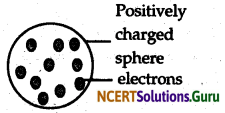• An atom consists of a positively charged into it.
• The negative and positive charges are equal in magnitude and hence, an atom is electrically neutral.

Question 7.
If in an element K shell is completely filled then what will be the
(a) number of valence electrons
(b) valence and
(c) name of element.
(a) Number of valence electrons = 2
(b) Valence = Zero
(c) Name of element = Helium

Question 8.
If chlorine atom is available in the form of say two isotopes 35C17(75%). 37Cl17(25%). Calculate the average atomic mass of bromine.
The average atomic mass of bromine would be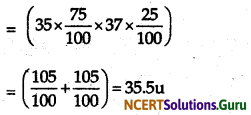Question 9.
The average atomic mass of a sample of an element X is 12.2 u. What is the percentage of isotopes 12X6 and 14X6 in the sample?
Atomic mass of X =12 unit
Let the percentage of 12X6 = X %
Percentage of 14X6 = Y% = (100 – x)%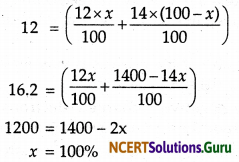Question 10.
What are isotopes? State their uses.
Isotopes of an element are the atoms which have the same atomic number: different atomic mass number because of the presence of the different number neutrons. These isotopes are used in

• Generation of electricity as fuels in nuclear power stations such as uranium-235.
• In chemotherapy such as Co-60 is used in treatment of cancer.
• Isotope of iodine is used in treatment of goitre.
• Research works to find the metabolic pathways.

Question 11.
How is an isotope different from an isobar?
Isotopes are the atoms of an element which have same atomic number – differ in the atomic mass number because they carry different number of neutrons but Isobars are the atoms of different elements which have same atomic mass number but they differ in their atomic number.

Question 12.
Which of the following are the isotopes and isobars? Why?
18X8, 18Y6, 16X8
18X8, and 18Y6 are isobars because they have different atomic number but same atomic mass number.
18X8, and 16X8 are isotopes because they have same atomic number but different atomic masses.

Question 13.
How is an alpha particle different from an atom of helium?
Alpha particle is the nucleus of helium represented by the formula without any electron but an atom of helium consists of 2 electrons along nucleus having two protons and neutrons.

Question 14.
Calculate the number of electrons, protons and neutrons in the following
40X20, 14Y6, 238Z92
40X20 – Number of protons = 20, Number of electrons = 20 Number of neutrons = 20
14Y6 – Number of protons = 6, Number of electrons = 6 Number of neutrons = 8
238Z92 – Number of protons = 92, Number of electrons = 92 Number of neutrons = 146

Question 15.
Why the valence electrons are considered responsible for the chemical activity of an element?
Valence electrons are the electrons present in the outermost shell of an atom. The number of these electrons determines that how many electrons an atom need to gain or lose, to gain stability. The lesser is the number of electrons required to be lost or gained, the reactive is the element.

Question 16.
How is a discharge tube different from an ordinary tube?
A discharge is a tube with the gas under very low pressure i.e. 1 atmospheric pressure where it is possible to generate the electric discharge but in an ordinary tube has average atmospheric pressure in which an electrical discharge cannot be produced in any condition.

Question 1.
What are cathode rays? How are they produced?
When an electric discharge is produced in a discharge tube at low pressure 10-2 atmospheric pressure and the voltage of 10,000 V, the rays emitted from the cathode are called cathode rays. These rays consist of negatively charge particles called electrons because they get deflected towards the positively charged electrode when passing through an electric field.In discharge tube, the low pressure is maintained to have less number of molecules hence, minimum interference in the path of the cathode rays emitted from cathode. The emission of these rays proved that the atoms are not indivisible as stated by Dalton in his atomic theory but contain negatively charge electrons.

Question 2.
What are anode rays? Describe their nature.
Anode rays are the positively charged rays produced in a discharge tube having a perforated cathode. These rays consist of positively charge particles called protons. These rays get deflected towards the negatively charged electrode when passing through an electric field.

The charge on a proton is equal to the charge on an electron, 1.6 × 10-19C but is resite in sign being positively charged.

Question 3.
What observations did Rutherford made in his alpha particles scattering experiment? What did he conclude from his observations?
Rutherford in his alpha particle experiment when he made the alpha particles incident on the surface of a very thin and fine gold foil observed that

• Most of the fast moving alpha particles passed through the gold foil.
• Some of the alpha particles underwent deflection in their path.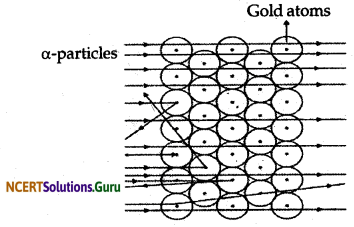• Very few i.e. one out of 12,000 alpha particle bounced back at 180°.
From his observations Rutherford concluded and proposed that
• There is a positively charged centre in an atom. He called this positively charged centre as nucleus.
• The size of the nucleus is very small as compared to the size of an atom.
• The negatively charged electrons are present outside the nucleus revolving around it.

Question 4.
Explain Rutherford’s α-particle scattering experiment and give its observation and conclusion drawn.
Rutherford’s α-particle scattering experiment:
Fast moving α-particles were made to fall on a thin gold foil. Particles have + 2 charge and 4u mass, and considerable amount of energy.Observations:

• Most of the α-particles passed straight through the foil.
• Some Of the α-particles were deflected by small angles by the foil.
• One out of every 12000 particles rebounded.

Conclusion from observation:

• Most of the space inside the foil is empty.
• Positive charge of atom occupies very less space.
• Mass of the atom is concentrated in the centre with all positive charge concentrated in small volume within the atom.
error: Content is protected !!